Discovering the Intrinsic Cardinality and Dimensionality
of Time Series using Minimum Description Length

Supporting Material
• Slide to briefly explain our idea including all figures in higher resolution.

Intrinsic Cardinality and Dimensionality
Donoho-Johnstone Benchmark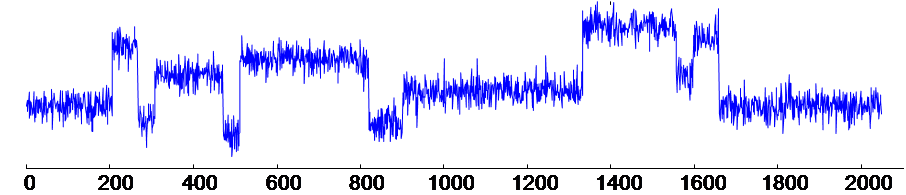• This well-known benchmark is created ten years ago and cited by many publications.
• We also provide the mirror of the time series in txt file here. Note that this file is required to run the codes for figure 9, 10, and 11.
• Source code for generating the result in figure 9.   Matlab figure behind figure 9. (Find an intrinsic dimensionality)
• Source code for generating the result in figure 10. Matlab figure behind figure 10. (Find an intrinsic cardinality)
• Source code for generating the result in figure 11. Matlab figure behind figure 11. (Compare among three models)

Physiology Dataset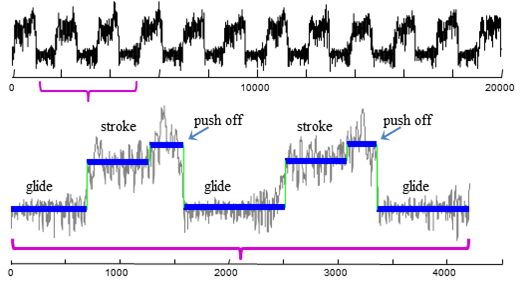• Above time series is an excerpt data from the Muscle dataset in .
• Original data is in the author's webpage; we also provided the mirror of the data here.
• Source code for generating the result in figure 13.
• Matlab figure to show the result in figure 13.

Ourlier Detection
Astronomy Dataset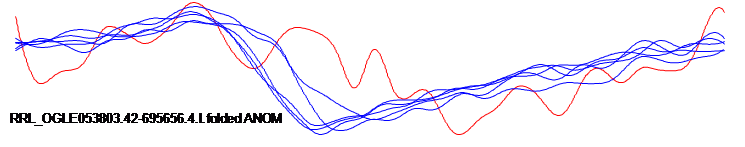• Six of 5,327 star light curves time series in class RRL annotated anomalies by human from .
• The mirror of the dataset with labels can be downloaded here.
Cardiology Dataset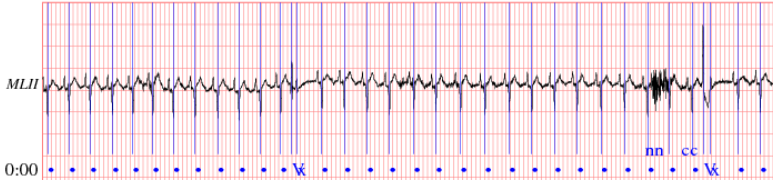• A cardiology data from PhysioBank ATM of the MIT BIH Arrhythmia dataset, record 108, signal MLII.

Compare to L-method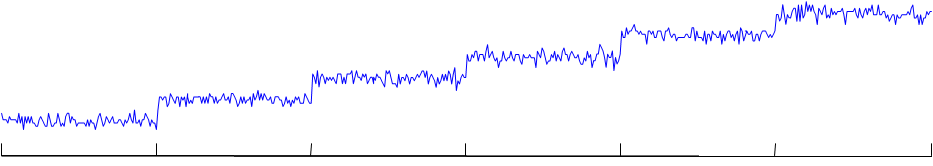• The time series are easily generated by step function with noise. Time series of six steps is shown in figure above.
• We compare our method with L-method; The comparison result shows that our method can find the correct dimensionality in every case.
• We tested on 2-step time series to 16-step time series. These are the results for our method and L-method.

Source Code
• Code for discovering an intrinsic dimensionality of  APCA model. (Fix cardinality)
• Code for discovering an intrinsic dimensionality of  PLA model. (Fix cardinality)
• Code for discovering an intrinsic dimensionality of  DFT model.(Fix cardinality)
• Code for discovering an intrinsic cardinality of  APCA model. (Fix dimensionality)

References:
•  Mörchen, F. and Ultsch, A. 2005. Optimizing time series discretization for knowledge discovery. KDD, 660-665.
•  Protopapas, P., Giammarco, J. M., Faccioli, L., Struble, M. F., Dave, R., and Alcock, C. 2006. Finding outlier light-curves in catalogs of periodic variable stars. Monthly Notices of the Royal Astronomical Society, 369 (2006), 677–696.

Contact Information: rakthant "at" cs "dot" ucr "dot" edu